# Algebra 1 : How to find mode

## Example Questions

### Example Question #101 : How To Find Mode

Find the mode of the following data set: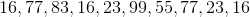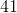Explanation:

Find the median of the following data set:Whenever we are working with a data set, it can be helpful to put the terms in order: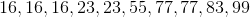Now that our terms are in order, we can do all sorts of things with them.

In this case, we need the mode.

The mode is simply the most repeated term in the sequence.

With our terms in order it should be pretty clear that our mode is 16

### Example Question #102 : How To Find Mode

Find the mode of the following set: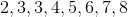Explanation:

To find the mode of any set, find the number which occurs the greatest number of times in the set.  The best answer is:### Example Question #102 : How To Find Mode

Find the mode of the following set: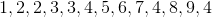Explanation:

To find the mode of any set, find the number which occurs the greatest number of times in the set.  The best answer is:### Example Question #104 : How To Find Mode

Find the mode of the following set: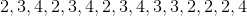Explanation:

To find the mode of any set, find the number which occurs the greatest number of times in the set.  The best answer is:### Example Question #105 : How To Find Mode

Find the mode of the following set: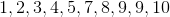Explanation:

To find the mode of any set, find the number which occurs the greatest number of times in the set.  The best answer is:### Example Question #103 : How To Find Mode

Find the mode: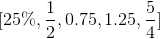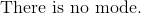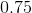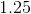Explanation:

The mode is defined as the numbers that have the highest frequency.

The numbers will need to be converted to a common form.  Let's choose decimal.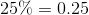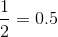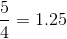Rewrite the dataset.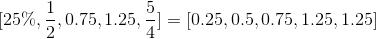We will notice that 1.25 has the highest frequency.

The answer is:### Example Question #107 : How To Find Mode

Find the mode of the following data set: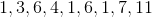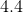Explanation:

The mode of a data set is the number that appears the most frequently.  Given the data set

1, 3, 6, 4, 1, 6, 1, 7, 11

the number that appears most frequently is 1.  Therefore, the mode is 1.

### Example Question #104 : How To Find Mode

Find the mode of the following dataset: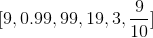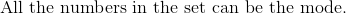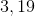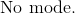Explanation:

The mode is the number or numbers with the highest occurrence in a dataset.

Notice that many numbers in the dataset share common numbers of nine, but each of the six numbers in the set are independent numbers.

There are no numbers in this dataset that have more than one occurrence, which means there is no mode.

The answer is:### Example Question #109 : How To Find Mode

Find the mode of the following data set:

4, 2, 7, 8, 8, 2, 8, 10, 7Explanation:

To find the mode of a data set, you determine which number appears most frequently.  Given the data set

4, 2, 7, 8, 8, 2, 8, 10, 7

the number 8 appears the most often (3 times).  Therefore, 8 is the mode.

### Example Question #110 : How To Find Mode

What is the mode of the following dataset?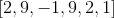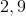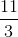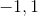Explanation:

The mode is the number or numbers with the highest frequency in the dataset.

Only the two and nine are repeating numbers and have more than one occurrence.

The other numbers only appear once and cannot be the mode.

Therefore, the answer is:### All Algebra 1 Resources